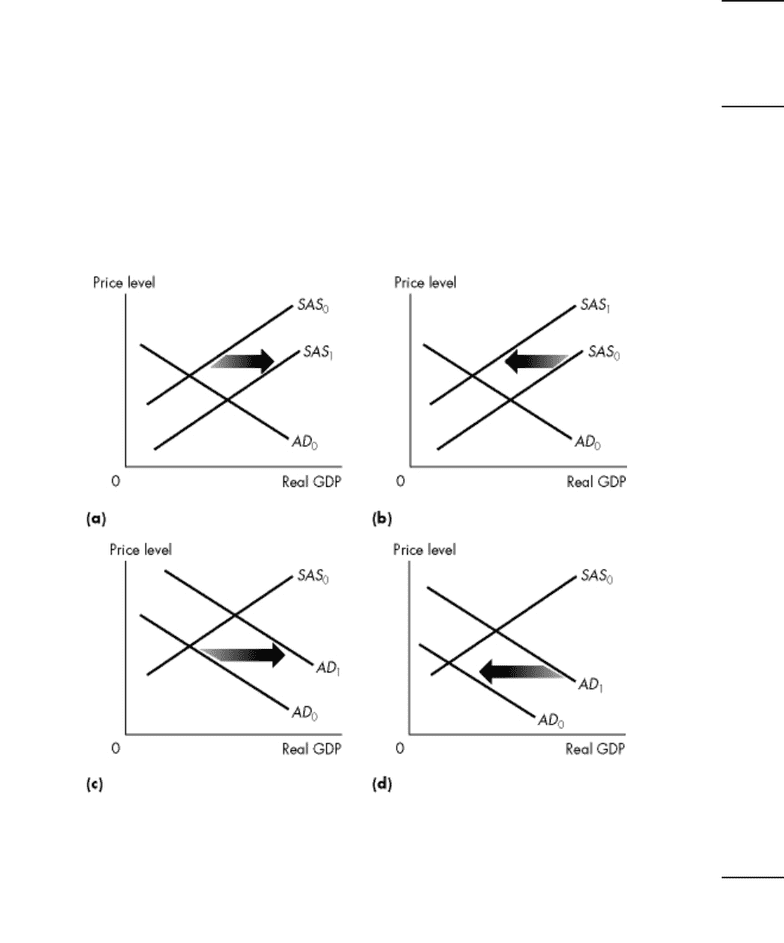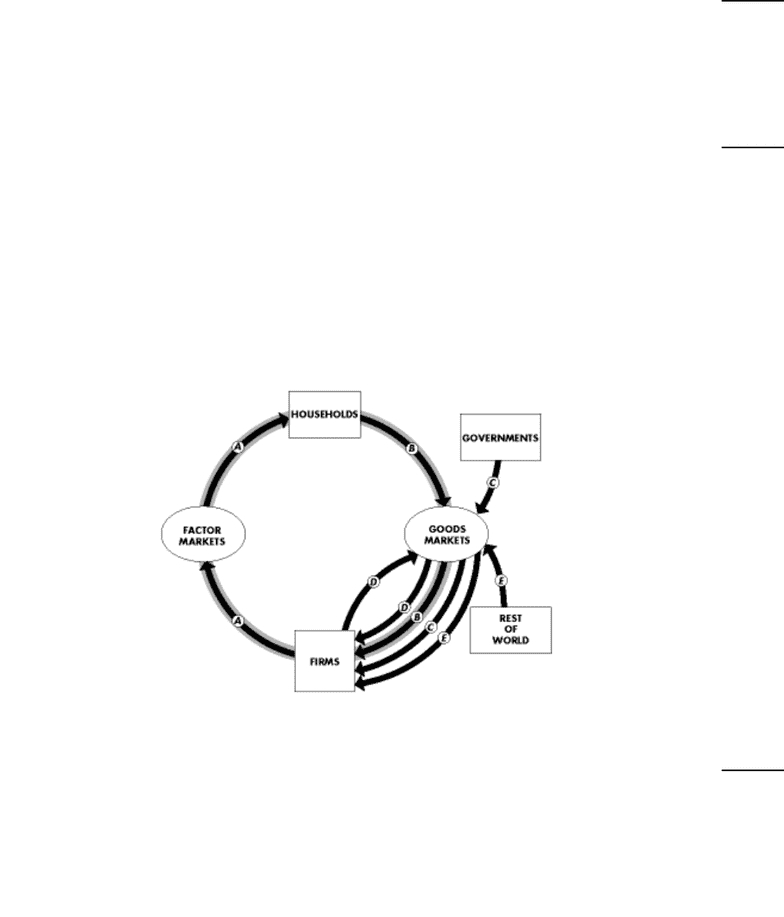Study Guides (390,000)
CA (150,000)
York (10,000)
ECON (900)
ECON 1010 (200)
All (70)
Midterm

# ECON 1010 Midterm: ECON1010M Midterm 2012 Winter BExam

Department
Economics
Course Code
ECON 1010
Professor
All
Study Guide
Midterm

This preview shows pages 1-3. to view the full 17 pages of the document.Exam
Name___________________________________
Macro Economics 1010.N Mid Term Test Winter 2012---TEST B
MULTIPLE CHOICE. Choose the one alternative that best completes the statement or answers the question.
1)
The fixed basket of Econoland consists of 10 units of A, 20 units of B, and 30 units of C. Current
prices are \$1 per unit of A, \$2 per unit of B, and \$3 per unit of C. Base year prices are \$1 for each
unit of A,B, and C. What is the CPI in the current year?
1)
A)
233
B)
43
C)
140
D)
430
E)
100
2)
A recession is a period with
2)
A)
positive growth rate in real GDP that lasts at least two quarters.
B)
negative growth rate in real GDP that lasts at least two quarters.
C)
negative growth rate in real GDP that lasts at least one quarter.
D)
a slowdown in real GDP growth but not necessarily negative real GDP growth.
E)
positive growth rate in real GDP that lasts at least one quarter.
Use the figure below to answer the following questions.
Figure 26.2.1
3)
Refer to Figure 26.2.1. Which graph illustrates what happens when government expenditure
decreases?
3)
A)
(a)
B)
(b)
C)
(c)
D)
(d)
E)
(a) and (b)
1

Only pages 1-3 are available for preview. Some parts have been intentionally blurred.4)
If investment increases by \$200, and as a result income increases by \$800, then the
4)
A)
multiplier is 3.
B)
slope of the AE curve is 1/4.
C)
slope of the AE curve is 0.75.
D)
multiplier is 1/4.
E)
none of the above.
5)
The aggregate expenditure curve and the aggregate demand curve are
5)
A)
linked because if the price level rises, the aggregate expenditure curve shifts downward, and
there is a movement down along the aggregate demand curve.
B)
the same curve, just with different names.
C)
not related at all.
D)
linked because if the price level rises, the aggregate expenditure curve shifts downward, and
the aggregate demand curve shifts leftward.
E)
linked because if the price level rises, the aggregate expenditure curve shifts downward, and
there is a movement up along the aggregate demand curve.
Use the figure below to answer the following questions.
Figure 20.1.1
6)
Refer to Figure 20.1.1, which shows the circular flow of expenditure and income for Venus. During
2008, A was \$100, B was \$50, C was \$30 and D was \$10. How much is aggregate expenditure?
6)
A)
\$90.
B)
\$50.
C)
\$75.
D)
\$100.
E)
None of the above.
2

Only pages 1-3 are available for preview. Some parts have been intentionally blurred.7)
If the labor force participation rate is rising and the working-age population is not changing, then
the
7)
A)
size of the labor force is falling.
B)
number of unemployed people is falling and the size of the labor force is rising.
C)
number of unemployed people is rising and the size of the labor force is falling.
D)
number of employed people must be increasing.
E)
size of the labor force is rising.
Use the table below to answer the following question.
Table 27.1.1
The following table shows the relationship between consumption
expenditure (C) and disposable income (YD) for a hypothetical economy.
YD (dollars) C(dollars)
100
200
300
400
500
600
225
300
375
450
525
600
8)
Refer to Table 27.1.1. The marginal propensity to consume is
8)
A)
equal to 1 when YD equals \$600.
B)
0.25.
C)
1.33.
D)
increasing as YD increases.
E)
0.75.
3